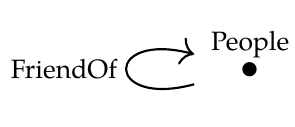> **Puzzle 122.** Let \$$\mathcal{C}\$$ be the free category on this graph:

>> Let's use this as a database schema. Draw an example of a database built using this schema. Mathematically it's a functor \$$F:\mathcal{C} \to \textrm{Set}\$$. But what does this mean in concrete terms? You can draw it as a table, like you would see in a spreadsheet.

\$\begin{array}{c|c} \text{People} & \mathrm{FriendOf} \\\\ \hline Alice & Bob \\\\ Bob & Alice \\\\ \vdots & \vdots \\\\ Stan & Tyler \\\\ Tyler & Stan \\\\ \vdots & \vdots \end{array} \$
This table has a symmetry that's not required of every functor \$$F : \mathcal{C} \to \mathbf{Set}\$$ Another perfectly fine answer would have been this:
\$\begin{array}{c|c} \text{People} & \mathrm{FriendOf} \\\\ \hline Alice & Bob \\\\ Bob & Bob \\\\ \vdots & \vdots \\\\ Stan & Bob \\\\ Tyler & Alice \\\\ \vdots & \vdots \end{array} \$
**Puzzle.** Can we change the category \$$\mathcal{C}\$$ to a category \$$\mathcal{C}^\prime\$$ so that functors \$$F : \mathcal{C}^\prime \to \mathbf{Set}\$$ are just databases of the sort Keith drew, with the kind of symmetry that his table has?